# Logarithm of a number

(diff) ← Older revision | Latest revision (diff) | Newer revision → (diff)to a baseThe degreeto which the number(the base of the logarithm) must be raised in order to obtain; it is denoted by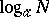; that is,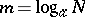means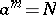. To every positive numberand given base,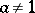, there corresponds a unique real logarithm (logarithms of negative numbers are complex numbers). The main properties of the logarithm are: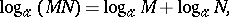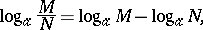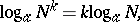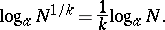These make it possible to reduce multiplication and division of numbers to the addition and subtraction of their logarithms, and the raising to powers and extraction of roots to the multiplication and division of the logarithm by the index of the power or root.

In accordance with the decimal system, the most commonly used are decimal logarithms, denoted by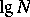. For rational numbers other than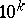withan integer, the decimal logarithms are transcendental numbers (cf. Transcendental number), which can be approximately expressed by finite decimal fractions. The integer part of a decimal logarithm is called the characteristic, and the fractional part is called the mantissa. Since, the decimal logarithms of numbers that differ by a multiple ofhave the same mantissa and differ only in the characteristics. This property is the basis for the construction of logarithm tables, which contain only the mantissa of the logarithms of integers.

The natural logarithms also have great significance; the base of these is the transcendental number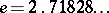; they are denoted by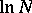. The transition from one base to another is carried out by the formula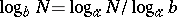; the factoris called the modulus of transition from the baseto the base. The formulas for the transition from natural logarithms to decimal logarithms and vice versa are: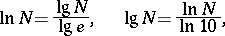Western writers of post-calculus mathematics almost always use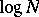instead ofto denote the natural logarithm (base) of. The numberis given by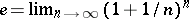and by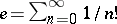(cf.(number)).
On the other hand, writers of calculus and pre-calculus books as well as manufacturers of calculators usually usefor the decimal (common) logarithm andfor the natural logarithm. Cf. also Logarithmic function.